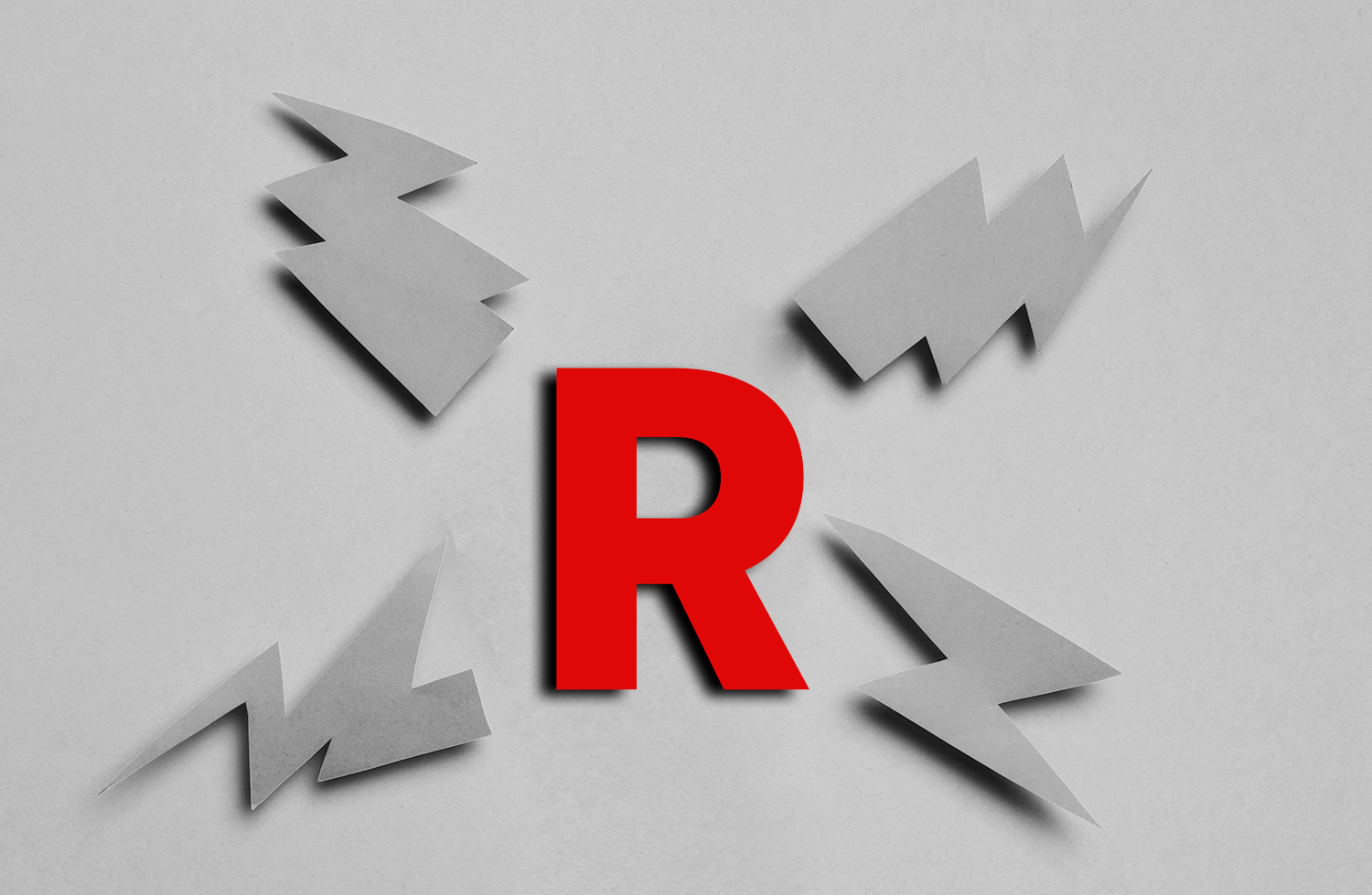##### Share
«  Blog
###### «  TRADING TO THE POWER OF “R”# TRADING TO THE POWER OF “R”

##### Oct 18, 2022

So how did your trading go today? And how does that compare with yesterday and every day last week and last month?

Some traders would answer those questions in terms of dollars gained and dollars lost. Others would measure their performance in percentages. Some might even think in terms of how their trades made them feel. Many, however, record each winning or losing trade in terms of an R-Multiple (with “R” standing for “risk”). They might talk about yearly returns as a percentage of their trading capital but do not assess individual trades in percentage terms.

## What Is An R-Multiple?

The concept of the R-multiple is the brainchild of professional trading coach, the late Dr. Van Tharp, who passed away on February 24, 2022. It is a way of measuring trade performance regardless of the prices of stock or even the amount of money won or lost. Tharp says that risk can be defined as the amount of money you are willing to risk in your trade if you are wrong about the position you’ve taken. This is called the initial Risk, or (R) for short.

Most successful traders know that it is absolutely essential to commit to at least two price points before they enter a trade: entry price and stop loss. The difference between these prices is the number of points risked on the trade. This is what’s called an R.

Many people seems to think of risk as something based on fear and associated with the probability of losing; for example, they might perceive being involved in futures or options as “risky,” or they may be overly optimistic about the trades they make because they don’t understand their worst-case risk or even think about such factors. They can be misled by trading terms like “options,” “arbitrage” and “naked puts.”

You must always have an exit point when you enter a position. The purpose of the exit point is to help you preserve your trading capital. It defines your initial risk (1R) in a trade.

For example, if you buy a stock at \$50 and have a stop loss at \$40, your initial risk is \$10 per share, meaning that 1R is equal to \$10. On the other hand, if you buy the same stock at \$50, but decide that you will get out of that trade if it drops to \$48, your initial risk is \$2 per share, and 1R is equal to \$2.

The goal of most traders using this system is never lose to more than 1R on any trade. However, there will be occasional cases that prices may slip through your stop loss and they will exceed 1R.

How would you measure your gains from a winning trade? Let’s assume a \$100 stock with a stop loss at \$10 goes up to \$120, where you exit and take profit. Your gain can be expressed as an R-Multiple of 2R because 1R was equal to \$10.

Van Tharp defines R-value as the initial risk taken in a given position, as defined by one’s initial stop loss.

In explaining R-multiples, he explains that all profits can be expressed as a multiple of the initial risk (R). For example, a 10-R multiple is a profit that is 10 times the initial risk. Thus, if your initial risk is \$10, then a \$100 profit would be a 10 R-multiple profit.

Here’s another example: Let’s say you buy a stock priced at \$500 and have a stop loss at \$480, meaning that 1R is equal to \$20. Then the stock’s price increases to \$540 and you take profit. You have taken \$40 or 2R profit. That’s the same as the last trade. But notice that this time the percentage increase in the stock’s price is quite different. 540-500=40 and (40/500)X100 = 8%.

You would have made the same amount of money on each trade, even though the percentage increases were quite different. This is because you keep 1R a consistent money amount; for example, 1R could equal 1% of your trading account.

## Total Risk

A common mistake is to think that a trader is risking the total dollar value of the trade, when the total risk is based on the position sizing and the stop loss for this trade.

So, let’s say you bought 100 shares at \$50 each with a stop loss at \$40, which would be 100 multiplied by the share cost of \$50 each, giving you a trade value or total cost of \$5,000. However, \$5,000 is not your risk for this trade, if you are willing to risk only \$10 per share, so \$10 multiplied by 100 shares = \$1,000 total risk for this position.

If you bought 100 of the same shares at \$50 each with a stop loss at \$48, your total cost would be \$5,000, but because you plan on getting out if the stock drops to \$48, your total risk is \$2 per share multiplied by 100 shares. In other words, you will be risking only \$200 of your \$5,000 trade.

In order to lose your full position of \$5,000, the shares you bought needs to go to zero, a very unlikely scenario.

Traders should know the expectancy of each individual trading strategy they are considering, they should especially know that if that number is a negative, they will lose money.

If they look at the results of 100 trades using the same strategy, they will have a long list of winners and losers. The number of winners compared to the number of losers is the win rate, which is generally expressed as a percentage.

In addition, they can calculate the expectancy of the strategy by adding up all the winners, subtracting the losers, and then dividing by the number of trades. For example: